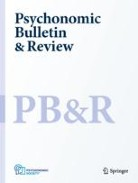## an introduction to MCMC sampling

Posted in Books, Kids, Statistics with tags , , , , , , , , , on August 9, 2022 by xi'anFollowing a rather clueless question on X validated, I had a quick read of A simple introduction to Markov Chain Monte–Carlo sampling, by Ravenzwaaij, Cassey, and Brown, published in 2018 in Psychonomic Bulletin & Review, which I had never opened to this day. The setting is very basic and the authors at pain to make their explanations as simple as possible, but I find the effort somehow backfires under the excess of details. And the characteristic avoidance of mathematical symbols and formulae. For instance, in the Normal mean example that is used as introductory illustration and that confused the question originator, there is no explanation for the posterior being a N(100,15) distribution, 100 being the sample average, the notation N(μ|x,σ) is used for the posterior density, and then the Metropolis comparison brings an added layer of confusion:

“Since the target distribution is normal with mean 100 (the value of the single observation) and standard deviation 15,  this means comparing N(100|108, 15) against N(100|110, 15).”

as it most unfortunately exchanges the positions of  μ and x (which is equal to 100). There is no fundamental error there, due to the symmetry of the Normal density, but this switch from posterior to likelihood certainly contributes to the confusion of the QO. Similarly for the Metropolis step description:

“If the new proposal has a lower posterior value than the most recent sample, then randomly choose to accept or
reject the new proposal, with a probability equal to the height of both posterior values. “

And the shortcomings of MCMC may prove equally difficult to ingest: like
“The method will “work” (i.e., the sampling distribution will truly be the target distribution) as long as certain conditions are met.
Firstly, the likelihood values calculated (…) to accept or reject the new proposal must accurately reflect the density of the proposal in the target distribution. When MCMC is applied to Bayesian inference, this means that the values calculated must be posterior likelihoods, or at least be proportional to the posterior likelihood (i.e., the ratio of the likelihoods calculated relative to one another must be correct).”

which leaves me uncertain as to what the authors do mean by the alternative situation, i.e., by the proposed value not reflecting the proposal density. Again, the reluctance in using (more) formulae hurts the intended pedagogical explanations.